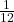## Determine la inercia rotacional de una varilla de 4 m de largo y 2 Kg de mesa si su eje de rotación esta situado a la mitad de la varilla.

Question

Determine la inercia rotacional de una varilla de 4 m de largo y 2 Kg de mesa si su eje de rotación esta situado a la mitad de la varilla.

in progress 0
6 months 2021-07-27T08:26:41+00:00 1 Answers 7 views 0

## Answers ( )

I = 2.667 kg m²

Explanation:

The moment of inertia of a body can be calculated by the expression

I = ∫ L² dm

For high symmetry bodies the expressions of the moment of inertia are tabulated, for a rod with its axis of rotation at its midpoint it is

I =m L²

let’s calculate

I =2  4²

I = 2.667 kg m²